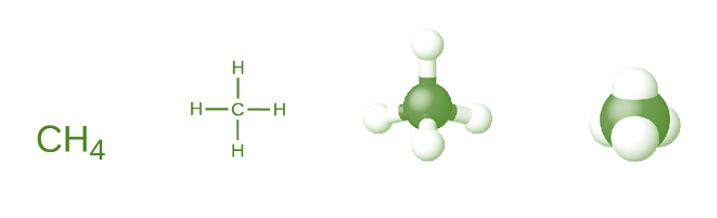GeeksforGeeks App
Open AppBrowser
Continue

# What is a Chemical Formula? Definition, Types, Examples

Atoms are tiny smallest particles of matter which can not be further divided is called an atom, which means that an atom is the smallest building block of matter. examples are Carbon (C), Hydrogen (H), Sodium (Na), etc. Atoms are combined using some set of rules the process of combination of two or more elements (Reactants) to form new compounds (product) is governed by certain laws called laws of chemical combination.

A chemical formula is a set of chemical symbols that are used to indicate the components contained in a compound as well as their proportions. Before we get into the details of constructing a chemical formula, we must first learn the fundamentals of chemical formulas. Let us proceed further!

### Molecular Formula

A molecular formula is a molecule representation that utilises chemical symbols to identify the types of atoms in the molecule, followed by subscripts to specify the number of atoms of each type in the molecule. (A subscript is used only when there are several atoms of the same kind present.) Molecular formulae are also used as abbreviations for compound names.

A compound’s structural formula contains the same information as its molecular formula (the kinds and quantities of atoms in the molecule), but it also indicates how the atoms are linked in the molecule. Methane’s structural formula includes symbols for one C atom and four H atoms, denoting the number of atoms in the molecule.Methane

The lines indicate the bonds that connect the atoms. (A chemical bond is an attraction that holds atoms or ions together in a molecule or crystal.) Later, we’ll go into chemical bonding and how to anticipate the arrangement of atoms in a molecule. For the time being, only know that the lines show how the atoms in a molecule are linked. A ball-and-stick model depicts the geometric arrangement of the atoms with non-scale atomic sizes, whereas a space-filling model depicts the relative sizes of the atoms.

### How to Write Molecular Formulae of Chemical Compounds

A binary compound is composed of two different elements only. Formulae of binary compounds can be written with the help of valencies of elements since both types of atoms forming the compound must lose, gain or share the same number of electrons.

Point be considered to write the molecular formula

• Determine the valency of the elements present in the compound.
• Write the chemical formula such that the sums of the valencies of the two elements are equal. Do this by determining the lowest common multiple of the two valencies.
• Always place metals first in the formula.

For example:

• Aluminium Oxide

The elements presents in Aluminium Oxide are Aluminium and Oxygen. Then,

The lowest common multiple of valencies is 6. Therefore,

Al = 2 × 3 = 6

O = 3 × 2 = 6

This implies that, the chemical formula is Al2O3

• Methane

The elements presents in Aluminium Oxide are Carbon and Hydrogen. Then,

The lowest common multiple of valencies is 4. Therefore,

C = 1 × 4

H = 4 × 1

This implies that, the chemical formula is CH4

• Calcium Oxide

The elements presents in Calcium Oxide are Calcium and Oxygen. Then,

The lowest common multiple of valencies is 2. Therefore.

Ca = 1 × 2

O = 1 × 2

This implies that, the chemical formula is CaO

### Sample Questions

Question 1: Calculate the number of electrons in Mg2+.

The Atomic number of Mg is 12.

2+ means loss of two electrons.

So, The atomic number of Mg2+ is 12 – 2 = 10

Hence, the number of electrons in Mg2+ is 10.

Question 2: Why do atoms form ions?

To achieve stability i.e. to complete its octet and attain stable electronic configuration near its noble gas atoms by losing or gaining electrons and thus acquire noble gas configuration.

Question 3: Give the difference between N2 and 2N.

In 2N, it means two molecules of a Nitrogen atom (N+N). N2 (two-atom of N) means one molecule of nitrogen gas.

2N does not show any bond, it just shows numbers of nitrogen whereas N2 shows that two nitrogen are bonded with a covalent bond, and it becomes nitrogen gas.

Question 4: Calculate the valency of (a) Mg (b) Ca (c)  Na and (d) Cl.

(a) Mg (Atomic number = 12) = distribution of electron in the orbits as (2, 8, 2)

Mg atom can lose two electrons to attain stability, so the valency of Mg is 2.

(b) Ca (Atomic number = 20) = distribution of electron in the orbits as (2, 8, 8, 2)

Ca atom can lose two electrons to attain stability, so the valency of Ca is 2.

(c) Na (Atomic number = 11) = distribution of electron in the orbits as (2, 8, 1)

Na atom can lose one electron to attain stability, so the valency of Na is 1.

(d) Cl (Atomic number = 17) = distribution of electron in the orbits as (2, 8, 7)

Cl atom can gain one electron to attain stability, so the valency of Cl is 1.

Question 5: Give the electronic configuration of the following:

(a) Al atom and its ion and

(b) O atom and its ion.

(a) The atomic number of Aluminium (Al) = 13.

Now, by losing three electrons it become Aluminium ion i.e, Al3+.

Therefore, the electronic configuration is given by:

(b) The atomic number of Oxygen (O) = 8.

Now, by gaining two electrons it become Oxygen ion i.e, O2-.

Therefore, the electronic configuration is given by:

My Personal Notes arrow_drop_up
Related Tutorials